# 混分统计

## 数学

### 选择题

1. 一条光线从点(﹣2,﹣3)射出，经y轴反射后与圆$(x+3)^2+(y﹣2)^2=1$相切，则入射光线所在直线的斜率为()A. $\frac{3}{2}或\frac{2}{3}$ B.$\frac{4}{3}或\frac{3}{4}$ C.$\frac{5}{3}或\frac{3}{5}$ D.$\frac{5}{4}或\frac{4}{5}$

• 分析：计算能力不足
2. 设m,n∈R，若直线$(m+1)x+(n+1)y-2=0$与圆$(x-1)^2+(y-1)^2=1$相切，则$m+n$的取值范围是()A.$[1-\sqrt3,1+\sqrt3]$ B.$(-\infty,1-\sqrt3]\cup[1+\sqrt3,+\infty)$ C.$[2-2\sqrt2,2+2\sqrt2]$ D.$(-\infty,2-2\sqrt2]\cup[2+2\sqrt2,+\infty)$

• 解法：由点到直线距离公式得$m+n=m\cdot n\le(\frac{m+n}{2})^2$，令$x=m+n,x+1\le \frac{x^2}{4}$解得$x\in[2-2\sqrt2,2+2\sqrt2]$
• 分析：对均值不等式变形使用不熟练，考试时慌张没把握好节奏。
3. 数学家欧拉1765年在其所著的《三角形几何学》一书中提出：任意三角形的外心、重心、垂心在同一条直线上，后人称这条直线为欧拉线．已知△ABC的顶点A(2,0)，B(0,4)，若其欧拉线的方程为x－y＋2＝0，则顶点C的坐标是() A. (－4,0) B. (0，－4) C. (4,0) D. (4,0)或(－4,0)

• 解法：设出点C的坐标，由重心坐标公式求得重心，代入欧拉线得一方程，求出AB的垂直平分线，和欧拉线方程联立求得三角形的外心，由外心到两个顶点的距离相等得另一方程，两方程联立求得点C的坐标。
• 分析：不记得三角形重心坐标$(\frac{x_a+x_b+x_c}{3},\frac{y_a+y_b+y_c}{3})$了，得好好巩固。画图可以看出选项，但填答案的时候忘记了。
4. 设有一组圆$C_k:(x-k+1)^2+(y-3k)^2=2k^4(k\in N^*)​$。下列四个命题，正确的有几个() ①存在一条定直线与所有的圆均相切； ②存在一条定直线与所有的圆均相交；③存在一条定直线与所有的圆均不相交； ④所有的圆均不经过原点。

• 解法：根据图象可知这些圆互相内含，不存在一条定直线与所有的圆均相切，不存在一条定直线与所有的圆均不相交，所以①③错;②圆心在$y=3(x+1)$上;④将(0,0)代入圆的方程，有$(-k+1)^2+9k^2-2k^4=0,10k^2-2k+1=2k^4$，左边为奇数右边为偶数故无满足要求k存在。（①③详解为求k,k+1的圆的位置关系不等式，可观察到使①③不成立k，略）
• 分析：有一定难度，但②④应该能够自行证出。

### 解答题

1. 设平面直角坐标系xOy中，设二次函数$f(x)=x^2+2x+b(x\in R)$的图象与两坐标轴有三个交点，经过这三个交点的圆记为C．求：

（Ⅰ）若b=﹣3求圆C的方程；

（Ⅱ）满足条件的b的取值范围；

（Ⅲ）问圆C是否经过某定点（其坐标与b无关）？请证明你的结论.

• 解法：(1)(3)使用一般式更为简便，$x^2+y^2+Ex+Dy+F=0$，令y=0结合二次函数得$\begin{cases}E=2\\F=b\end{cases}$，回代(0,b)得圆的方程，(3)随后用圆系方程解得定点。(2)注意x,y轴交点不重合故$b\ne0$。
• 分析：听讲不认真，用圆的一般式方程大大降低复杂度。
2. 已知⊙C过点P（1，1），且与$\odot M:(x+2)^2+(y+2)^2=r^2$关于直线x+y+2=0对称．

（Ⅰ）求⊙C的方程；

（Ⅱ）设Q为⊙C上的一个动点，求$\overrightarrow{PQ}\cdot\overrightarrow{MQ}$的最小值；

（Ⅲ）过点P作两条相异直线分别与⊙C相交于A，B，且直线PA和直线PB的倾斜角互补，O为坐标原点，试判断直线OP和AB是否平行？请说明理由．

• 解法：(1)基础题。(2)设出Q坐标，代入向量积式子，后用参数方程或者线性规划求解最值。(3)设出点斜式方程，与圆联立，得AB横坐标，后化简对比。
• 分析：第2问乱混乱写，连基本参数方程和线性规划都忘了使用，基础不牢。第3问讲过，~没时间没听讲也懒得算。

## 物理

1. 如图所示，质量为m、电荷量为+q的a粒子和质量为4m、电荷量为+2q的b粒子，经过同一电场同时由静止开始加速，然后经过同一偏转电场，最后打到荧光屏上，不计粒子所受的重力及粒子间的相互作用力，下列说法正确的是()A. 它们打到荧光屏上的同一点 B. 它们打到荧光屏上的不同点 C. a粒子比b粒子先到达荧光屏 D. 到达荧光屏上时，a粒子的动能小于b粒子的动能

• 解法：A：此类模型运动轨迹固定，与比荷无关（证明略）。C：$\varDelta E_k=\frac{1}{2}mv_x^2=W_E=U_1q$，计算得此项正确。D：$\varDelta_{total} E_k=U_1q+U_2q,~q_a\le q_b$故$E_{ka}\le E_{kb}$。
• 分析：基础知识遗忘过多了，得好好复习。
2. 如图甲所示，x轴上固定两个点电荷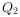位于坐标原点O），轴上有M,N,P三点，间距MN=NP ,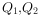在轴上产生的电势随x变化关系如图乙.下列说法错误的是()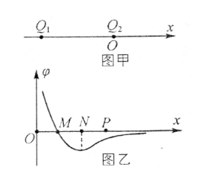A. M点电场场强大小为零B. N点电场场强大小为零 C. M,N之间电场方向沿x轴负方向 D. 一正试探电荷从P移到M过程中，电场力做功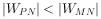• 分析：试利用微元法证明$\varDelta\phi\div\varDelta x=E$，图像性质有所遗忘，及时复习。

## 语文

1. 说明文阅读不够严谨，仍然对词义句义的调换不够细致。
2. 小说阅读自己不知所云，自评为0～2分，但在老师那里混到了6分。近来做题太少，完全不会读题意了，不会答题了，都答非所问。
3. 人物传记阅读又是答非所问，自评为1分实际得3分，需要加强知识与技巧的迁移（小说阅读方法“听其言观其行参其评”引申到传记阅读等）。
4. 文言文预期3分实际5分。实词忘了许多，赶快积累复习。
5. 诗词鉴赏0分，这才在意料之中。完全瞎答的，又是答非所问系列，对基础概念的定义不清楚，不懂什么叫“艺术技巧”。需要后期多多训练积累。
6. 基础词句题混得还行，成语题一如既往全靠蒙，病句题B没有完全搞清楚错在哪里，就是感觉不对，于是混分成功。
7. 作文玄学，周围的人都得分不高低于平时，我还维持了48分。原本以为立意（科研者应有品德）角度太低属于43分档，结果还有个48保底，又是玄学+运气。

## 化学

1. 下图是金属镁和卤素单质(X2)反应的能量变化示意图。下列说法正确的是( )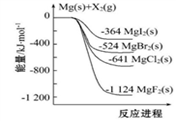A. 金属镁和卤素单质$X_2$具有的能量比$MgX_2$ 的高

D. 由图可知此温度下$MgBr_2(s)$与$Cl_2(g)$反应的热化学方程式为：$MgBr_2(s)+Cl_2(g)=MgCl_2(s)+Br_2（g）ΔH=-117kJ·mol^{－1}$

• 当时看不出D有什么地方错了，也不太确定A哪里错了。没想到化学老师做的时候也很懵逼。A的错误也许在于没有写条件、状态、物质的量。
2. 向绝热恒容密闭容器中通入$SO_2$和$NO_2$，一定条件下使反应$SO_2(g)+NO_2(g)\rightleftharpoons SO_3（g)+NO(g)$达到平衡，正反应速率随时间变化的示意图如图所示．由图可得出的正确结论是（　　）D. △t1=△t2时，$SO_2$的转化率：a～b段小于b～c段

• 排除了ABC，然后看不懂D什么意思，就选了D。可能的意思是a～b段$\alpha(SO_2)$都小于c～d段，或者$\varDelta_{ab}\alpha(SO_2)\le\varDelta_{cd}\alpha(SO_2)$，但不管哪种D都是对的。还是太naive了，看不懂题目。
3. 在中和热测定实验中存在用水洗涤温度计上的盐酸溶液的步骤，若无此操作步骤，则测得的中和热____(填“偏大”、“偏小”或“不变”)。

• 瞎猜的偏小。当时完全没往影响起始温度方面想，以为测最终温度时因为$NaOH$过量末温度不变。结果是因为会让起始温度升高因此热量偏小导致中和热数值偏小。
4.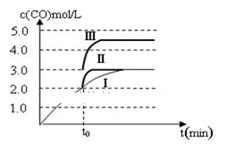一定温度下，在3 L容积可变的密闭容器中发生反应b:$CO_2(g)＋H_2(g)\rightleftharpoons CO(g)＋H_2O(g)~\varDelta H_2$，已知c(CO)与反应时间t变化曲线Ⅰ如图3所示，若在$t_0$时刻分别改变一个条件，曲线Ⅰ变为曲线Ⅱ和曲线Ⅲ。当曲线Ⅰ变为曲线Ⅱ时，改变的条件是 当曲线Ⅰ变为曲线Ⅲ时，改变的条件是 。

• 第二个空当时犹豫了一下还是只填了增压。此类数值变化关系明确的一般要求答出详细变化。